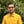Trusted answers to developer questions
Trusted Answers to Developer Questions

Related Tags

d
communitycreator

# What is the ceil() function in D?Harris Amjad

Grokking Modern System Design Interview for Engineers & Managers

Ace your System Design Interview and take your career to the next level. Learn to handle the design of applications like Netflix, Quora, Facebook, Uber, and many more in a 45-min interview. Learn the RESHADED framework for architecting web-scale applications by determining requirements, constraints, and assumptions before diving into a step-by-step design process.

### Overview

The ceil() function returns the nearest smallest integer value that is greater than or equal to a number.

The figure below shows the mathematical representation of the ceil() function:

Mathematical representation of ceil() function

Note: We need to import std.math in our code to use the ceil() function. We can import it by using:
import std.math

### Syntax

ceil(number)
// number should be float, double or real.


### Parameter

This function requires a number as a parameter.

### Return value

ceil() returns the nearest, smallest integer value greater than or equal to a number sent as a parameter.

Note: - If -0.0, real.infinity, -real.infinity, real.nan, or -real.nan is sent as a parameter then it returns the parameter as it is.

### Example

The code below shows the use of the ceil() function in D:

import core.stdc.stdio;import std.stdio;//header required for functionimport std.math;int main(){    //integer    writeln ("The value of ceil(4.0) : ",ceil(4.0));    //positive double value    writeln ("The value of ceil(2.56) : ",ceil(2.56));    //negative double value    writeln ("The value of ceil(-2.56) : ",ceil(-2.56));    //exceptional outputs    writeln ("The value of ceil(-0.0) : ",ceil(-0.0));    writeln ("The value of ceil(real.infinity) : ",ceil(real.infinity));    writeln ("The value of ceil(-real.infinity) : ",ceil(-real.infinity));    writeln ("The value of ceil(real.nan) : ",ceil(real.nan));    writeln ("The value of ceil(-real.nan) : ",ceil(-real.nan));    return 0;}
Using the ceil() method

### Explanation

In the code above:

• Line 4: We add the header std.math required for ceil() function.

• Line 9: We calculate the ceiling of the integer value using ceil().

• Line 12: We calculate the ceiling of the positive double value using ceil().

• Line 15: We calculate the ceiling of the negative double value using ceil().

• Lines 19 to 23: We calculate the ceiling of exceptional numbers using ceil().

RELATED TAGS

d
communitycreator

CONTRIBUTORHarris Amjad

Grokking Modern System Design Interview for Engineers & Managers

Ace your System Design Interview and take your career to the next level. Learn to handle the design of applications like Netflix, Quora, Facebook, Uber, and many more in a 45-min interview. Learn the RESHADED framework for architecting web-scale applications by determining requirements, constraints, and assumptions before diving into a step-by-step design process.

Keep Exploring

Learn in-demand tech skills in half the time